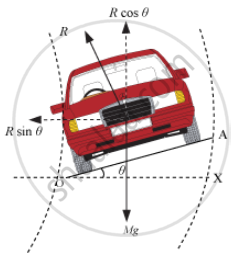Share

# Draw a Diagram Showing All Components of Forces Acting on a Vehicle Moving on a Curved Banked Road. - Physics

#### Questions

Draw a diagram showing all components of forces acting on a vehicle moving on a curved banked road.

Draw a neat labelled diagram showing the various forces and their components acting on a vehicle moving along curved banked road

#### Solution

• Motion of a car on a banked road:

For the vehicle to go round the curved track at a reasonable speed without skidding, the greater centripetal force is managed for it by raising the outer edge of the track a little above the inner edge. It is called banking of circular tracks.Consider a vehicle of weight Mg, moving round a curved path of radius r, with a speed v, on a road banked through angleθ.

The vehicle is under the action of the following forces:

• The weight Mg acting vertically downwards

• The reaction R of the ground to the vehicle, acting along the normal to the banked road OA in the upward direction

The vertical component R cos θ of the normal reaction R will balance the weight of the vehicle and the horizontal component R sin θ will provide the necessary centripetal force to the vehicle. Thus,

R cosθ = Mg …(i)

R sinθ = (Mv^2)/r .......(ii)

On dividing equation (ii) by equation (i), we get

(R sin theta)/(R cos theta) = (Mv^2//r)/Mg

tan theta = v^2/(rg)

As the vehicle moves along the circular banked road OA, the force of friction between the road and the tyres of the vehicle, F = μR, acts in the direction AO.

The frictional force can be resolved into two components:

• μ R sinθ in the downward direction

• μ R cosθ in the inward direction

Since there is no motion along the vertical,

R cos θ = Mg + μ R sinθ ……. (iii)

Let vmax be the maximum permissible speed of the vehicle. The centripetal force is now provided by the components R sinθ and μ Mg cosθ, i.e.,

R sin θ + μ R cosθ = (Mv_max  ^2)/r .....(iv)

From equation(iii),we have

Mg = R cosθ (1−μ tanθ)…(v)

Again from equation (iv), we have

(Mv_max  ^2)/r

= R cosθ (μ + tanθ) …(vi)

On dividing equation (iv) by (v), we have

(v_max ^2)/(gr) = (µ + tan theta)/(1 - tan theta)

=> v_max = [(g r (µ  + tan theta)/(1 - µ tan theta)]^(1//2)

Maximum optimum speed depends on:
1) Radius of the curved path,
2) Coefficient of friction
3) angle on inclination
Regards

Is there an error in this question or solution?